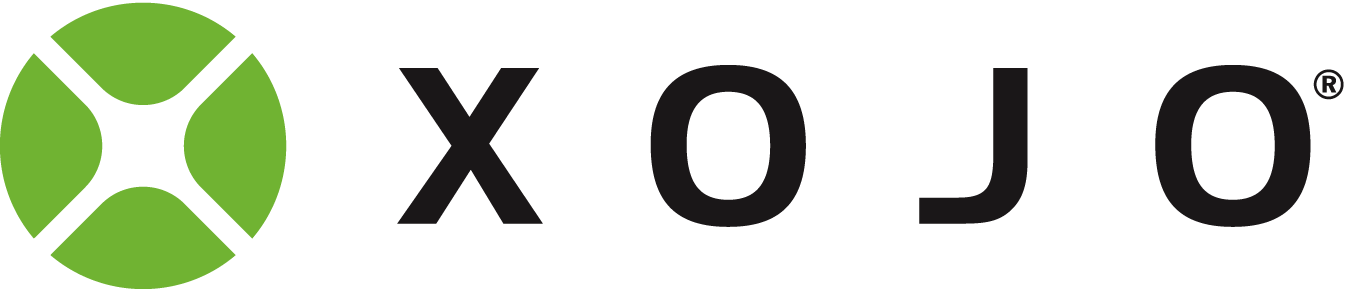# How to round technically correct

Hello,

I’m currently having an issue with rounding. I have a currency value. I want to round this financially correct. The problem is, that Round, Floor and Ceil don’t offer what I need or I don’t understand how it should work.

For example, when I have the number 2,34 and I calculate the taxes, this number could as well be 2,3451. The last two digits in this case, the third and fourth decimal are past the middle. This means it should be rounded up. But not to the next full number but too 2,35.

``````Format (Round((nettoWithTax* 1.16) + (NettoLoweredTax* 1.05) + taxFreeNumber), "-###,##0.00")
``````

This would output a totally wrong number, since it will always round up or down to the fullest. How can I do this instead?

Edit: The number is rounded by in this case the third decimal. 1,0072 would be 1,01 in two digits.

Greetings,

If you need to round to two decimal places, then first multiply the number by 100, then round it, and finally divide by 100:
RoundNumber = round(myNumber * 100)/100

2 Likes